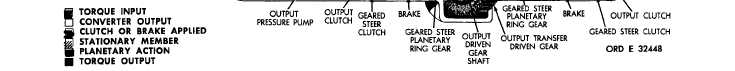Figure   29.   First-gear,   full-right-steer   torque   path,   converter   operation
Custom SearchC H A P    2,   SEC   V T O R Q U E     P A T H S P A R   5   3 Figure   29.   First-gear,   full-right-steer   torque   path,   converter   operation 53.   TORQUE   PATH - F I R S T    G E A R, FULL-RIGHT    STEER,    CONVERTER OPERATION   (fig.   29) .   T o r q u e    i s    t r a n s m i t t e d    t o    t h e    s t e er planetary   ring   gears   in   the   same   manner   as that   for   first   gear,   as   described   in   par.   47a a n d   ,   above.   From   this   point,   to   the   power train   outputs,   the   torque   paths   for   the   right and   left   sides   are   different. .   T h e    c l u t c h - b r a k e    m e t h o d    i s    u s e d    f or f i r s t - g e a r     s t e e r .     S t e e r i n g     r e s u l t s     w h e n     t he brake   is   applied   at   one   side   while   the   drive c l u t c h    i s    e n g a g e d    a t    t h e    o p p o s i t e    s i d e .    T he d r i v e    c l u t c h    i s    t h e    g e a r e d    s t e e r    c l u t c h .    T he vehicle   steers   toward   the   braked   side. .   In   right   steer,   in   first   gear,   the   right 5 6 brake   is   applied   while   the   right-drive   clutch is   released.   The   drive   clutch   on   the   left   side is   still   engaged   and   continues   to   drive.   Hy- draulic   action,   controlled   by   the   steer   control v a l v e ,    r e l e a s e s    t h e    d r i v e    c l u t c h    a n d    a p p l i es the   brake.   An   opposite   movement   of   the   steer control   valve   will   release   the   left-geared   steer clutch   (the   drive   clutch),   apply   the   left   brake, and   result   in   left   steer. .   In   full   steer,   the   applied   brake   locks the   steer   planetary   carrier   and   rotation   stops a t    t h a t    s i d e .    I n    p a r t i a l    s t e e r ,    t h e    a p p l i ed b r a k e    o n l y    s l o w s    t h e    s i d e    t o w a r d    w h i c h    t he t u r n    i s    m a d e .    T h e    d e g r e e    o f    s t e e r    i s    c o n- trolled   by   the   degree   of   movement   of   the   steer c o n t r o l .    R e f e r    t o    T A B U L A T E D    D A T A ,    p a r. 7,   for   the   gear   ratios   which   apply   to   first   gear, full-right  steer.Integrated Publishing, Inc. - A (SDVOSB) Service Disabled Veteran Owned Small Business